# ML Wiki

## Coverability Graph

This is a way of representing states of workflows, similar to Reachability Graph

### Motivation

Consider the following YAWL flow:

•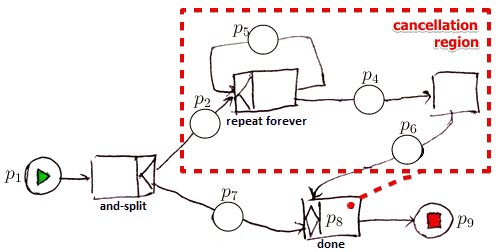.
• because of the Cancellation Regions the Reachability Graph of this workflow becomes infinitely large
• it is possible to know when we need to stop expanding it?
• if there was no Cancellation Region, it would be enough just to repeat it 2 times to see that there's no proper termination
• but in this case we don't know when to stop
• $\Rightarrow$ can no longer use it for checking for Workflow Soundness

However there is a concept of Coverability Graphs that are

• computationally less expensive
• can still be used to decide on some properties

### Coverability

Let's consider the following Petri Net:

•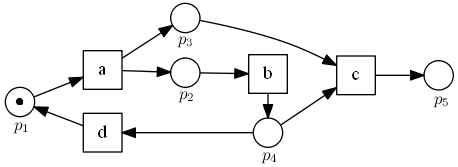• how we can represent $\infty$ many nodes in this reachability graph?
•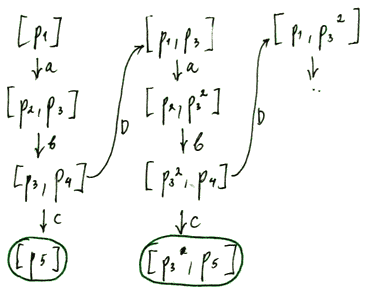• note that marking $[p_1, p_3] < [p_1]$ - strictly larger
• thus with marking $[p_1, p_3]$ the petri net can do all the same as $[p_1]$ plus a little bit more
• so if it's possible to get from one marking $M_1$ to another marking $M_2$ s.t. $M_2$ covers $M_1$ completely - then we have a loop

Coverability:

• marking $M$ is coverable by $M'$ $\iff$
• there $\exists$ a marking $M'$ s.t. $M \to^* M'$ and $M \leqslant M'$

Let's construct the coverability graph for this example

•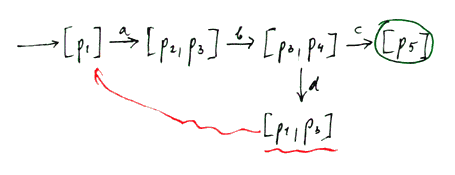• look at all the nodes that you can reach
• if you notice some marking that covers another marking, add a loop to the coverability graph
•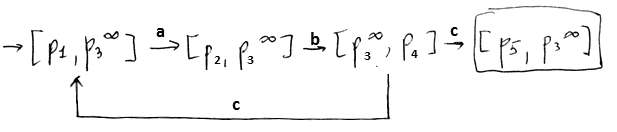• note that this graph is finite
• and we can see that in this graph we do have the option to complete